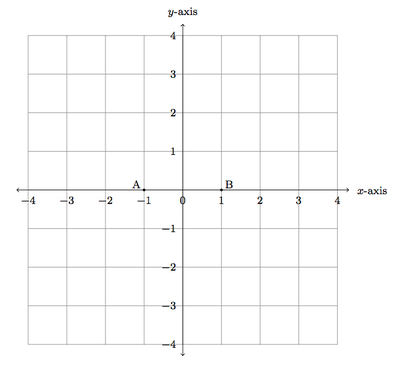# Triangles inscribed in a circle

Alignments to Content Standards: G-GPE.B.5

Suppose $A = (-1,0)$ and $B = (1,0)$ are points in the coordinate plane as pictured below:Suppose $C = (x,y)$ is a third point in the plane, not on the $x$-axis. Show that $\angle ACB$ is a right angle if and only if $(x,y)$ lies on the circle $x^2 + y^2 = 1$.

## IM Commentary

The goal of this task is to use ideas about linear functions in order to determine when certain angles are right angles. The key piece of knowledge implemented is that two lines (which are not vertical or horizontal) are perpendicular when their slopes are inverse reciprocals of one another. This algebraic relationship is related to the geometric fact that angle $C$ of a triangle $ABC$ is a right angle exactly when $C$ lies on the circle with $\overline{AB}$ as diameter. This task examines and develops this relationship.

In the task $A = (-1,0)$ and $B = (1,0)$, making the algebra relatively straightforward. The same argument will work for any pair of points $A, B$ in the plane but this will require more algebraic work along the lines of G-GPE.1. If the teacher wishes to work on this standard, she may choose some other pairs of points for $A$ and $B$. Alternatively, a similarity transformation can be chosen to take a given pair of points $P$ and $Q$ to $(-1,0)$ and $(1,0)$: these transformations preserve circles and angle measurements and so the general case is reduced to the one considered here.

The argument presented here can be profitably compared and contrasted with the more classical one covered in ''Right Triangles Inscribed in Circles I, II.'' The argument here using the coordinate system and slopes of lines is much simpler. Students should be familiar with both approaches but this provides an example where the use of coordinates is very powerful. The teacher may need to provide some guidance for how the students should get started on this task, perhaps by choosing one or two explicit points for $C$ and leading them through the calculations of slopes in the solution for these specific points.

It is vital in this task for students to attend to precision in their reasoning: the algebraic formulas used to derive that $C$ is on the circle with $\overline{AB}$ as diameter only make sense under certain conditions and special care is needed to make sure that these conditions are met in all situations where $C$ is on the circle but not on the $x$-axis. Because guidance is likely necessary and because part of its value lies in its connection to other arguments, this task is recommended for instructional purposes only.

## Solution

Below is a picture of a point $C$ not on $\overleftrightarrow{AB}$:If $C = (x,y)$ then we have

\begin{align} \text{slope}\left(\overleftrightarrow{AC}\right) & = \frac{y}{x+1}\\ \text{slope}\left(\overleftrightarrow{BC}\right) &= \frac{y}{x-1} \end{align}

Looking at these expressions, we see that special care is needed for the case $x=\pm 1$, so we dispose of these cases first: Since $C$ is not on the $x$-axis, the case that $x=\pm 1$ implies that $C$ is not on the circle, and also that $\angle ACB$ is not a right angle. This verifies the claim for the special case of points with $x=\pm 1$, so we can assume that $x \neq \pm 1$ from now on. Lines $\overleftrightarrow{AC}$ and $\overleftrightarrow{BC}$ are perpendicular when their slopes satisfy the relation $$\text{slope}\left(\overleftrightarrow{AC}\right) = \frac{-1}{\text{slope}\left(\overleftrightarrow{BC}\right)}.$$ Substituting the values above, we see that $\overleftrightarrow{AB}$ and $\overleftrightarrow{BC}$ are perpendicular exactly when $$\frac{y}{x+1} = \frac{1-x}{y}.$$ Note that these expressions both make sense because $x \neq -1$ and $y \neq 0$ since otherwise $A, B,$ and $C$ would be collinear and $ABC$ would not be a triangle. This means that $y^2 = 1-x^2$, or equivalently, $x^2 + y^2 = 1$. So angle $C$ is a right angle in triangle $ABC$ precisely when $C$ lies on the circle (but not on the $x$-axis) with $\overline{AB}$ as diameter.

We emphasize here that the argument of the previous paragraph can be run in both directions: if $C$ is on the circle $x^2 + y^2 = 1$ then $\angle C$ is a right angle and if $\angle C$ is a right angle then $x^2 + y^2 = 1$. The key is that the equation $x^2 + y^2 = 1$ is equivalent to the equation $$\frac{y}{x+1} = \frac{1-x}{y}$$ when $y$ is not zero and $x$ is not plus or minus 1: $C$ lies on the unit circle when the first equation holds while the rays forming this angle are perpendicular when the second equation holds. So $\angle C$ is a right angle if and only if point $C$ lies on the unit circle (with non-zero $x$ coordinate).